## Year 3 mental arithmetic: sets 33 and 34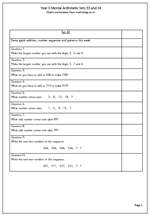This week’s mental arithmetic for year 3 looks at place value, number sequences and patterns as well as some quick addition.

One of the early questions asks what’s the largest number that can be made from the digits 2, 5 and 4. This is partly an ordering skill, making sure the largest digit is in the hundreds, second largest in the tens and smallest in the units. Some children find it quite difficult to retain three digits in their head and then manipulate them.

To find the next number in a sequence requires several skills. Firstly to listen carefully to the numbers, then to work out the pattern or rule and as to what is happening from one number to the next and thirdly to work out what the next number will be. These questions are kept at a fairly simple level and are all addition patterns.

There are also a couple of ‘odd number’ questions to check that children know about odd numbers.

Year 3 mental arithmetic: (sets 33 and 34)

## Year 4 maths worksheet on ordering decimal fractions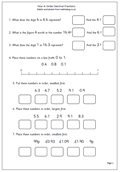One of the most important concepts in maths is to understand that the value of a digit depends on its place in the number – otherwise we could only possibly have 9 numbers and zero! So the digit 2 in 120 has a different value than the 2 in 210. The 2 in 120 has a value of 20, whilst the 2 in 210 has a value of 200.

By Year 4 children should be applying this idea to decimals. The first column to the right of the decimal point is tenths so the 1 in 2.1 has a value of one tenth and the 1 in 2.01 has a value of one hundredth. This is best shown with money: one penny is £0.01, or one hundredth, whilst 10p is £0.10 or one tenth of a pound. (It is also important that we always put two digits after the decimal when using money, but not necessarilyat other times.)

This maths worksheets looks at both place value and ordering simple decimal fractions, using the concepts above.

year 4 worksheet: Order decimal fractions

## KS2 Maths SAT Paper B question 6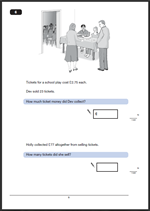Question 6 of the KS2 maths SAT Paper B is in two parts and it would be most sensible to use a calculator.

The first part of the question looks at the cost of 23 tickets at £2.75 each.

The answer to question 6a is  £63.25
One mark awarded for a correct answer.
Any unambiguous indication of the correct amount is acceptable, including: £63.25p, £63.25 pence or £63,25.

Suggested method:

This question is mainly a matter of deciding what process to carry out and using a calculator accurately.
Some children have very little practice with a calculator and may be tempted not to use it.
Recognising that this is a multiplication question and carefully keying in the correct amount, including the decimal point should ensure a correct answer is reached.
Remind children it is always worth carrying out a reverse operation to check that the answer is correct. In this case £63.25 divided by 23 will give £2.75.

The second part gives the total amount from ticket sales and asks how many tickets were sold.

The answer to question 6b is 28.
One mark awarded for a correct answer.

Suggested method:

Easy one mark use a calculator to divide £77 by 2.75. Perhaps the one major error that children can make is to put the numbers in the wrong way round on the calculator (2.77 ÷ 77).

Why not visit ks2-maths-sats.co.uk for free SATs papers and a great SAT revision programme?

Question 6

Question 6 answers and suggested method 2011 paper B

## Year 3 maths challenge: how many ways to make 18?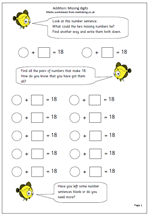Here is a worksheet that makes children think a little bit more. It shows a number sentence with two numbers that total 18. It asks what the two numbers could be.

The first thing to look for with this is children who use a logical or methodical approach. Usually children will write down the first answer that comes into their heads. That is why I have provided two blank number sentences at the top of the page. However, once they have done this they should start to revise their thinking and try to approach the task in an order so that they will know when they have reached all the possible answers.

This activity can be extended  using different numbers eg 19 or 20 and it is a useful exercise in helping children learn these pairs of numbers ‘off by heart’.

it can also be extended by allowing halves, which makes it quite a bit harder.

## Year 3 mental arithmetic: sets 31 and 32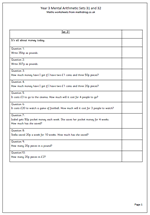This week’s mental arithmetic for year 3 is all on money! One important concept that need checking is converting pence to pounds.

For example 356p written in pounds is £3.56.

Watch out for £3.56p which you often see in shops, markets etc but which is incorrect. it is either £3.56 pounds or 356p.

Other questions include adding up totals of coins and working out word problems to do with money.

Remember if reading these questions out to read them clearly and slowly, repeating at least once. There are two sets of questions, but they can also be used to create further questions of your own along similar subjects and difficulty.

Year 3 mental arithmetic sets 31 and 32

## Telling the time in Year 2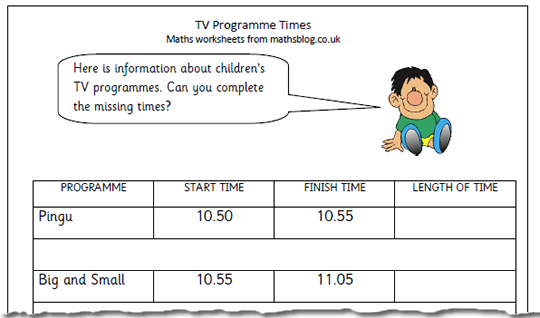Time is one of the most searched for subject areas on the site. By the end of year 3 children are expected to read the time on a 12 hour digital clock, and to the nearest 5 minutes on an analogue clock. They are also expected to be able to calculate time intervals and find start and finishing times for a given time interval.

This worksheet looks at working out the times of TV programmes and how long they last. Working within units of 5 minutes much of this is straightforward. However working out the start times is a little more tricky and children need to be confident with telling the time to 5 minutes.

Newspapers and TV programme listings are a really good source of material for work on time and can also result in a lot of discussion about favourite programmes etc. They can also be used as a data source for graph work.

This page and others can be found in the Year 2 Measuring category.

TV programmes: telling the time

## KS2 Maths 2011 Paper B: Question 5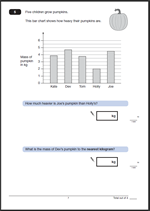Here we have question 5 from the KS2 maths 2011 Paper B. There are two parts to the question, both based on interpreting a block graph.

The answer to 5a is:   2.5 or 2 1/2

One mark awarded for a correct answer.

Suggested method:

This is a straightforward graph question which should prove to be an easy mark to gain.

The important part of this question is to look carefully at the scale showing the mass of the pumpkin in kilos. Every other line is marked in kilos, so every other line must be a half kilo.

Joe’s pumpkin has a mass of 4.5 kg. Holly’s has a mass of 2 kg. The difference is 4.5 kg – 2 kg = 2.5 kg.

Note the use of mass rather than weight in this question. Children should be very familiar with this.

The answer to 5b is: 5 kg

One mark awarded for a correct answer.

Suggested method:

This is a rounding question. The first thing to do is to look at the mass of Dev’s pumpkin. It is between 4.5 kg and 5 kg. As it is more than 4.5 kilos the mass should be rounded up to 5 kg (to the nearest whole kilogram).

Question 5 from Paper B 2011

Question 5 answers and suggested method 2011 Paper B

Why not visit ks2-maths-sats.co.uk for free SATs papers and a great SAT revision programme?

## Year 5 subtraction: missing digits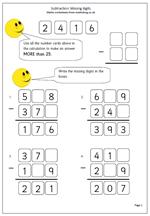Last week I published a page of addition with missing digits. This proved very popular and I have had several requests for something similar for subtraction; so here it is.

The calculations have been laid out in the tradition, standard method of written subtraction, except that two of the digits are missing. It is not as easy as it looks to find the missing digits, especially with a question such as:

41? – 2?9 = 207

In this question the units have to be adjusted, which will have a knock on effect in the tens. This page should only be given to children who have quite a good grasp of the standard written method of subtraction, but beware, it is harder than it looks!

Subtraction: missing digits

## Year 3 mental arithmetic: sets 29 and 30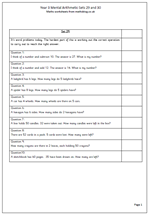This week the questions are all in the form of word problems. Generally children find these harder than just ‘sums’ because they are not told what operation needs to be carried out to reach the correct answer. Indeed, sometimes it can be tricky. Looking at the question,

‘I think of a number and subtract 10. The answer is 27. What is my number?’

Immediately the word subtraction will come to mind as it is used in the question. But, in fact, an addition has to be done to find the correct answer.

Several questions also require a good knowledge of the 2x, 5x and 10x tables.

If you are reading these out for children to answer be sure to read each question slowly and at least twice. The first time the question is heard the child will probably be thinking about what needs to be done. The second time reinforces the numbers involved.

Year 3 mental arithmetic (sets 29 and 30)

## Year 2 number challenge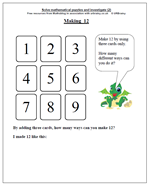Open ended questions are an excellent way to reinforce mathematical concepts, practice number skills and find out about some of the amazing patterns in maths. This maths worksheet is posted in our Year 2 category, under Using and Applying Maths and has proved to be one of our most popular resources.

The problem is to find as many ways as possible to make a total of 12 using three of the numbers on the cards. (Cards cannot be used twice in the same total so 4, 4 and 4 would not be allowed.)

It would be a good idea to have some cards printed with the digits on so that they can be cut out to help with this. They are available in the reception maths worksheets section.

Plenty of practice here at adding three small numbers. Also encourage working in a logical order and setting out results in a clear, logical way. There is no reason why recordings cannot be made of totals that are not 12.

Again plenty of opportunity for talking about the numbers and what counts as a different way. Is 1 + 2 + 9 the same as 2 + 1 + 9 ?

Make 12